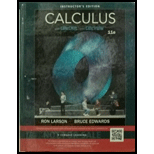Chapter 3.8, Problem 36E### Calculus (MindTap Course List)

11th Edition
Ron Larson + 1 other
ISBN: 9781337275347

#### Solutions

Chapter
Section### Calculus (MindTap Course List)

11th Edition
Ron Larson + 1 other
ISBN: 9781337275347
Textbook Problem

# Approximating Reciprocals Use the result of Exercise 35 to approximate (a) 1 3 and (b) 1 11 to three decimal places.

(a)

To determine

To calculate: The value of 13 up to three decimal places.

Explanation

Given:

The number is 13

Formula Used:

To calculate the value of 13 to three decimal places, use the formula,

xn+1=xn(2axn)

Calculation:

In order to find the value of 1a, use the approximation formula as,

xn+1=xn(2axn).

Now, assume x1=0.3, such that for n=1, the value of x2 would be,

x2=x1(23x1)=0.3(23(0.3))=0.33

For n=2, the value of x3 would be,

x3=x2(23x2)=0

(b)

To determine

To calculate: The value of 111 to three decimal places.

### Still sussing out bartleby?

Check out a sample textbook solution.

See a sample solution

#### The Answers to Your Study Problems

Solve them all with bartleby. Boost your grades with guidance from subject experts covering thousands of textbooks. All for just \$9.99/month

Get As ASAP

## Additional Math Solutions

#### Find more solutions based on key concepts